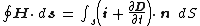# Displacement Current

(redirected from Maxwell displacement current)

## Displacement current

The name given by J. C. Maxwell to the term ∂ D /∂t which must be added to the current density i to extend to time-varying fields the magnetostatic result of A. M. Ampère that i equals the curl of the magnetic intensity H . In integral form this result is given by the equation below,where the unit vector n is perpendicular to the surface dS. The concept of displacement current has important consequences for insulators and for free space where i vanishes. For conductors, however, the difference between the above equation and Ampère's result is negligible. See Maxwell's equations

If one defines current as a transport of charge, the term displacement current is certainly a misnomer when applied to a vacuum where no charges exist. If, however, current is defined in terms of the magnetic fields it produces, the expression is legitimate.

McGraw-Hill Concise Encyclopedia of Physics. © 2002 by The McGraw-Hill Companies, Inc.
The following article is from The Great Soviet Encyclopedia (1979). It might be outdated or ideologically biased.

## Current, Displacement

In establishing the theory of the electromagnetic field, J. C. Maxwell proposed a hypothesis— later confirmed by experiment—stating that a magnetic field is created not only by the motion of charges (conduction current, or simply current) but also by any change in the electric field over time. Maxwell designated as “displacement current” a quantity equal to the change over time t of the induction D—or, more accurately, the quantity (∂D/∂t)/4π. The rotational magnetic field is determined by the total current j = jcond + (∂D/∂t)/4π, where jcond is the conduction current density. A displacement current creates a magnetic field according to the same law that applies to a conduction current; it is for this reason that the designation “current” is applied to the quantity (∂D/∂t)/4π. (SeeMAXWELL’S EQUATIONS.)

The Great Soviet Encyclopedia, 3rd Edition (1970-1979). © 2010 The Gale Group, Inc. All rights reserved.

## displacement current

[dis′plās·mənt ‚kə·rənt]
(electromagnetism)
The rate of change of the electric displacement vector, which must be added to the current density to extend Ampère's law to the case of time-varying fields (meter-kilogram-second units). Also known as Maxwell's displacement current.
McGraw-Hill Dictionary of Scientific & Technical Terms, 6E, Copyright © 2003 by The McGraw-Hill Companies, Inc.
Site: Follow: Share:
Open / Close